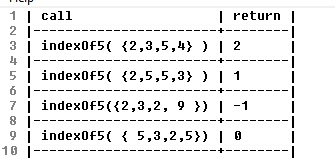# Array Fun 2 

Complete all the methods below. When you are done, copy and paste the testmethods.v4  into your class and run it to see if you have any obvious errors.

int sumEveryN(int[] nums, int n)

Description: This method returns the sum of every `n` elements of `num`s..

 Method Call return value/output sumEveryN( {1 , 2 , 3 , 4 }, 2 ) 4( ie 1 +3) sumEveryN( {13 , 42, 15, 33 , 44 , 16 , 52} ,3) 98 ( ie 13 + 33+ 52)

String[] doubleArr(String[] strs)

Description: This method returns a new version of `strs` in which each element now appears twice. This can be done with a for-each loop, which I believe is easier and more intuitive.

 Method Call return value/output doubleArr( {“a”,”b”,”c”} ) {“a”,”a”,”b”,”b”, “c” , “c”} doubleArr( {“math”,”ware”,”house”,”.com” }) {“math”,”math”,”ware”,”ware”,”house”,”house”,”.com”, “.com”}

int  indexOf5(int[] nums )

Description:This returns the index of the first occurrence element 5  or -1 if 5 does not appear anywhere in the array.

 Method Call return value/output indexOf5( { 2 , 3 , 5 , 4 } ) 2 indexOf5( { 2 , 3 , 5 , 4, 5  } ) 2 indexOf5( { 2 , 3 ,7  , 4, 3,   } ) -1

More Sample calls and return valsint indexOf(int[] nums, int num)

Description: This method returns the index value of the first appearance of `num` or -1 if `num` is not an element of `nums` .

 Method Call return value/output indexOf( {6,4 ,7,3, 4 }, 4) 1 indexOf( {6,4 7 ,3,2,7}, 7) 2 indexOf( {6,4 ,2,3}, 22) -1

int[] randos(int start, int end, int howMany)

Description:  This method returns an array of random numbers between [start,end] . Note make sure that each element in the new array attempts to make a new random int.

More example calls and returns :

double meanBetween(int[] nums, int min, int max)

Description:  This method returns the mean of nums ; however, this method only counts values within the range (min,max) as shown in the examples below:

int secondSmallest(int[] nums )

Description: This method returns the element of `nums` with the second smallest value.

Note: You may not modify the input array. For instance, you may not put nums in order,  which would be bad because you were not asked to modify the array.

@precondition: nums.length >= 2

Note: You will lose credit if you use a constant to represent the smallest or second smallest number. See pseudocode

 Method Call return value/output secondSmallest( { 2 , 18 , 22, 4 , 6 } ) 4 secondSmallest( { 3 , 7 , 15 , 1 ,101} ) 3

The question below is extra credit .
** int[] add(int[] num1, int[] num2, int base) throws ArithmeticException
@precondition `1 < base < 11`
@precondition `num1.length = 5 and num2.length = 5`
@postcondition: the returned array has 5 elements representing the sum or an ArithmeticException is thrown

Description: This method attempts to replicate addition. Consider both num1 and num2 represent the five digits of a number. Each element in the array stores one of the digits. For instance, the number 143 would be represented as {0,0,1,4,3 }. Numbers can be in any base between 2 and 10 inclusive , and the number 101102 in binary would appear in an arrays as : {1, 0, 1 , 1, 0 } . Let’s assume the numbers are positive. You should return an array representing the digits of the sum of num1 and num2.  You may not do any math besides addition. You may not use any external libraries for any math or base conversions.   If the number of digits in the sum exceeds the maximum number of digits (5) , you should throw an arithmetic exception as shown in the code below:

Note: You may not use any kind of helper or utility methods that are built into pre-defined Java classes for converting numbers between bases. Only use techniques taught in this class.

 Method Call return value/output add( {0,0,0 ,4,2},{0,0,0,5,1}, 10) {0, 0, 0, 9, 3} ie (42 + 51 = 93) add( {0, 0, 0 ,7,2},{0,0,0,5,1}, 10) {0, 0 , 1 , 2, 3} ie (72 + 51 = 123) add( {0, 0 , 0 , 1 , 1}, { 0 , 0 , 0 , 1 }, 2) {0, 0 , 1 , 0 , 0} ie ( 112 + 12= 1002 )

Old versions:

Array Fun 1

Array F un 2 (v 1)

Array Fun 3 (resizing included)